# MetroPCS LTE 9:16 PM 9 webassign.net Object A has mass mA-7 kg and initial momentum A<18,-6, 0> kg m/s, just before it strikes object B, which has mass mB 10 kg. Just before the collision...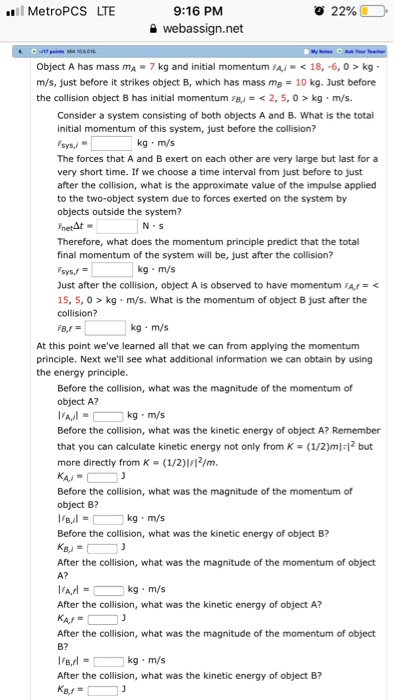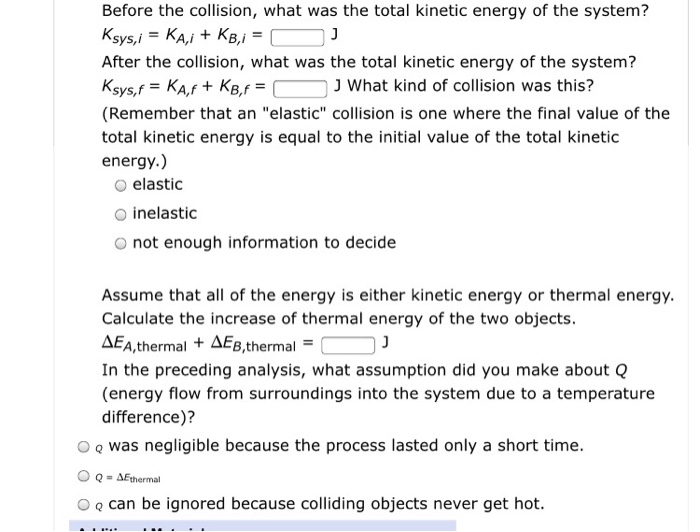MetroPCS LTE 9:16 PM 9 webassign.net Object A has mass mA-7 kg and initial momentum A kg m/s, just before it strikes object B, which has mass mB 10 kg. Just before the collision object B has initial momentum B 2, 5, 0> kg m/s. Consider a system consisting of both objects A and B. What is the total initial momentum of this system, just before the collision? lys,- The forces that A and B exert on each other are very large but last for a very short time. If we choose a time interval from just before to just after the collision, what is the approximate value of the impulse applied to the two-object system due to forces exerted on the system by objects outside the system? netAt Therefore, what does the momentum principle predict that the total final momentum of the system will be, just after the collision? sys,f Just after the collision, object A is observed to have momentum FAr kg m/s. What is the momentum of object B just after the collision? kg m/s N S At this point we've learned all that we can from applying the momentum principle. Next we'll see what additional information we can obtain by using the energy principle. Before the collision, what was the magnitude of the momentum of object A? kg m/s Before the collision, what was the kinetic energy of object A? Remember that you can calculate kinetic energy not only from K-(1/2)m 12 but more directly from K (1/2)I2/m Before the collision, what was the magnitude of the momentum of object B? kg m/s Before the collision, what was the kinetic energy of object B? After the collision, what was the magnitude of the momentum of object A? kg m/s After the collision, what was the kinetic energy of object A? After the collision, what was the magnitude of the momentum of object B? kg m/s After the collision, what was the kinetic energy of object B?
Before the collision, what was the total kinetic energy of the system? After the collision, what was the total kinetic energy of the system? J What kind of collision was this? (Remember that an "elastic" collision is one where the final value of the total kinetic energy is equal to the initial value of the total kinetic energy.) O elastic O inelastic O not enough information to decide Assume that all of the energy is either kinetic energy or thermal energy. Calculate the increase of thermal energy of the two objects ΔΕΑ,thermal + ΔΕΒ,thermal- In the preceding analysis, what assumption did you make about Q (energy flow from surroundings into the system due to a temperature difference)? O o was negligible because the process lasted only a short time. O o can be ignored because colliding objects never get hot.

according to the chegg rule we have to answer only four sub parts at a time.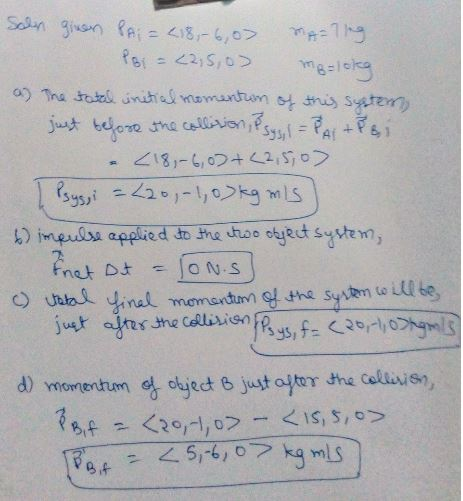##### Add Answer of: MetroPCS LTE 9:16 PM 9 webassign.net Object A has mass mA-7 kg and initial momentum A<18,-6, 0> kg m/s, just before it strikes object B, which has mass mB 10 kg. Just before the collision...
Similar Homework Help Questions
• ### Object A has mass mA = 9 kg and initial momentum PA = < 15,-8,0 >...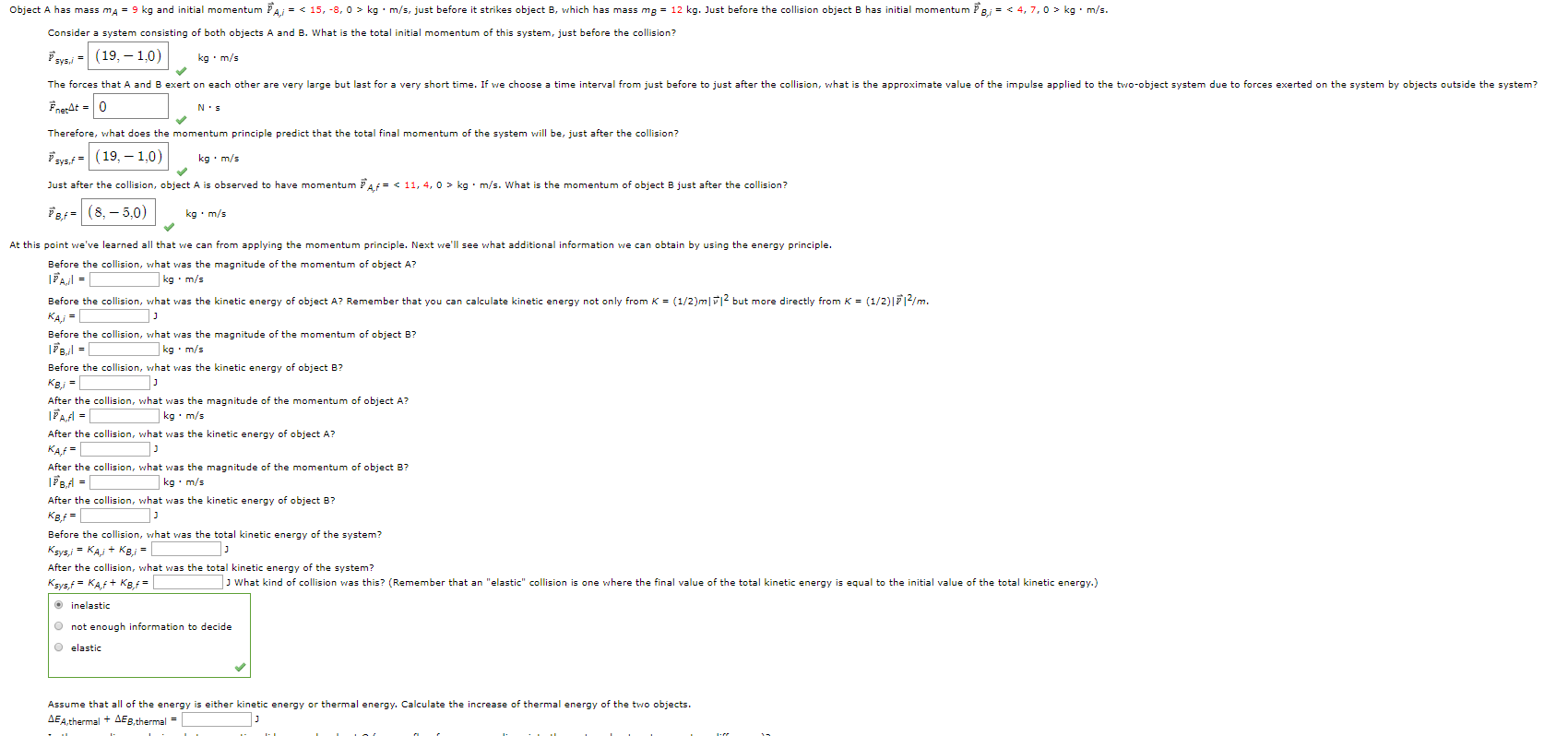Object A has mass mA = 9 kg and initial momentum PA = < 15,-8,0 > kgm/s, just before it strikes object B, which has mass mg = 12 kg. Just before the collision object B has initial momentum 8,1 = < 4,7,0 > kg. m/s. Consider a system consisting of both objects A and B. What is the total initial momentum of this system, just before the collision? sysj = (19,-1,0) kg.m/s The forces that A and B exert...

• ### Big Momentum promblem, 1st right answer gets lifesaver rating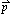Object A has mass mA = 7 kg and initial momentum A,i = < 15, -8, 0 > kg· m/s, just before it strikes object B, which has massmB = 10 kg. Justbefore thecollision object B has initial momentumB,i =<6, 7, 0> kg · m/s.Consider a system consisting of both objects Aand B. What is the total initial momentum of this system, justbefore the collision?sys,i = <, , 0 > kg · m/sThe forces that A and B exert on...

• ### kg m/s hits a nomentum of the ball is(5 wall and bounces straight back with the...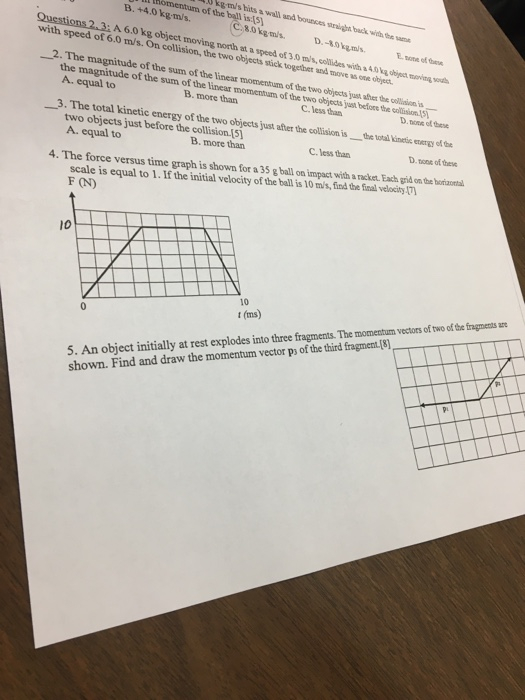kg m/s hits a nomentum of the ball is(5 wall and bounces straight back with the sam B. +4.0 kg-m/s C8.0kgm/s. Questions 2, 3; A 6.0 kg object moving north at a speed of 3.0 m/s, collides with a 4.0kg objot moving sous D.-8.0kgm/s Enone of the with speed of 6.0 m/s. On collision, the two objects stick togetber and move s one cbject 2. The magnitude of the sum of the linear momentum of the two objects just aher...

• ### A6.65 kg bowling bal moving at 10.0 m/ s colides with a 1.60 kg bowling pin, scattering It with a...A6.65 kg bowling bal moving at 10.0 m/ s colides with a 1.60 kg bowling pin, scattering It with a speed of 8.00 m/s and at an angle of 36.0% with respect to the initial direction of the (a) Calculate the final velocity (magnitude in m/s and direction in degrees velocity (magnitude in m/s and direction in degrees counterclockwise from the original direction) of the bowling ball magnitude m/s direction counterdlockwise from the original direction of the bowling ball (b)...

• ### In outer space rock 1 with mass 9 kg and velocity <3400, -3100, 3400> m/s, struck...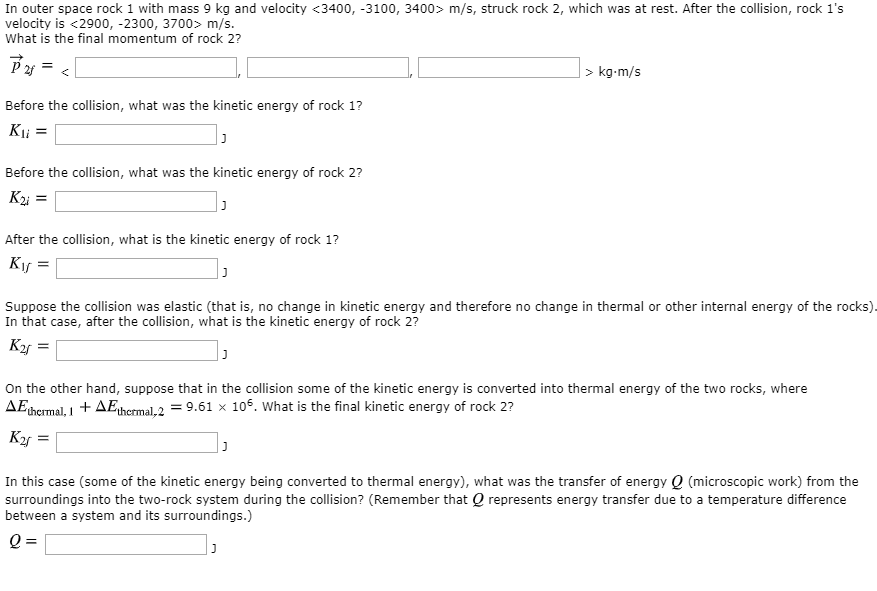In outer space rock 1 with mass 9 kg and velocity <3400, -3100, 3400> m/s, struck rock 2, which was at rest. After the collision, rock 1's velocity is <2900, -2300, 3700> m/s. What is the final momentum of rock 2? P 2 = > kg-m/s Before the collision, what was the kinetic energy of rock 1? Kli= Before the collision, what was the kinetic energy of rock 2? K2 = After the collision, what is the kinetic energy of...

• ### 1. Two asteroids collide head-on and stick together. Before the collision, asteroid A (mass 1,000 kg)...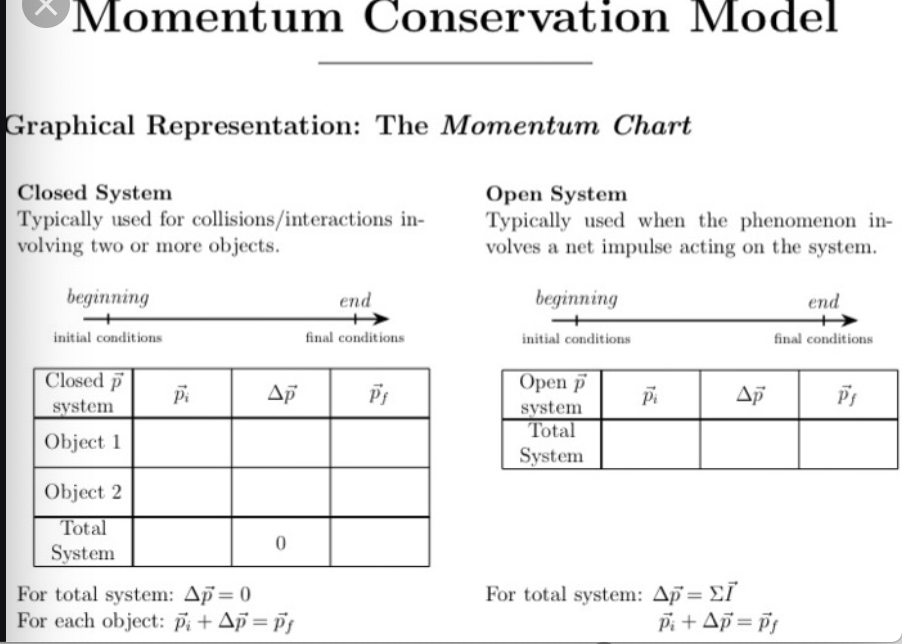1. Two asteroids collide head-on and stick together. Before the collision, asteroid A (mass 1,000 kg) moved at 100 m/s and asteroid B (mass 2,000 kg) moved at 80 m/s in the opposite direction. Use momentum conservation (make a complete Momentum chart) to find the velocity of the asteroids after the collision. 2. Two asteroids identical to those in (1) collide at right angles and stick together. "Collide at right angles" means that their initial velocities were perpendicular to each...

• ### A car of mass 2400 kg collides with a truck of mass 4600 kg, and just...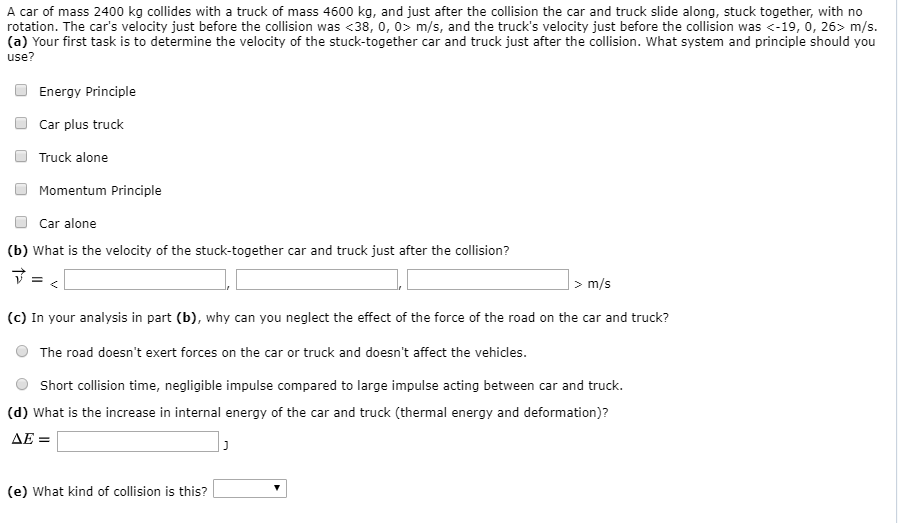A car of mass 2400 kg collides with a truck of mass 4600 kg, and just after the collision the car and truck slide along, stuck together, with no rotation. The car's velocity just before the collision was <38, 0, 0 m/s, and the truck's velocity just before the collision was C-19, 0, 26> m/s. (a) Your first task is to determine the velocity of the stuck-together car and truck just after the collision. What system and principle should you...

• ### ** An object of mass m1 =7.1 kg moving at 5.3 m/s strikes a stationary second...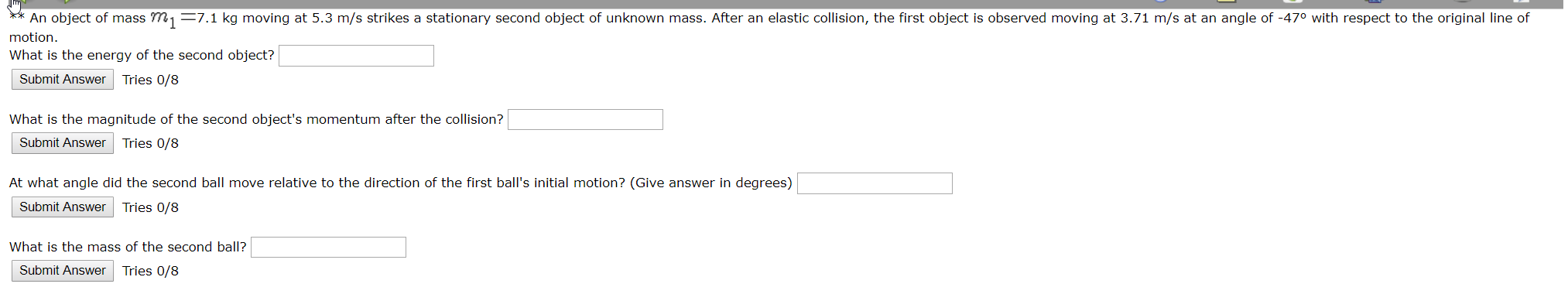** An object of mass m1 =7.1 kg moving at 5.3 m/s strikes a stationary second object of unknown mass. After an elastic collision, the first object is observed moving at 3.71 m/s at an angle of -47° with respect to the original line of motion. What is the energy of the second object? Submit Answer Tries 0/8 What is the magnitude of the second object's momentum after the collision? Submit Answer Tries 0/8 At what angle did the second...

• ### Problem 1: An object with mass m = 2 kg slides down a frictionless incline that...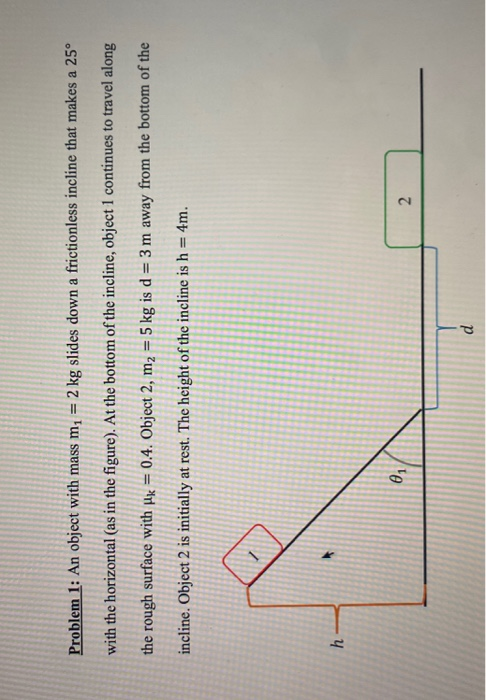Problem 1: An object with mass m = 2 kg slides down a frictionless incline that makes a 25° with the horizontal (as in the figure). At the bottom of the incline, object 1 continues to travel along the rough surface with Hk = 0.4. Object 2, m2 = 5 kg is d = 3 m away from the bottom of the incline. Object 2 is initially at rest. The height of the incline is h = 4m. h 0...

• ### Problem 1: An object with mass m = 2 kg slides down a frictionless incline that...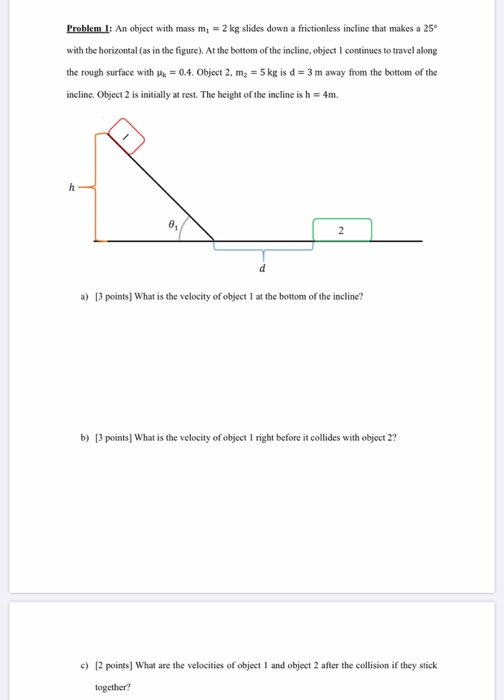Problem 1: An object with mass m = 2 kg slides down a frictionless incline that makes a 25° with the horizontal (as in the figure). At the bottom of the incline, object I continues to travel along the rough surface with Hk = 0.4. Object 2, m2 = 5 kg is d = 3 m away from the bottom of the incline. Object 2 is initially at rest. The height of the incline is h = 4m. h 2...

Free Homework App